# Polarization of Light from Basics to InstrumentsPage 1

#### WATCH ALL SLIDES

Slide 1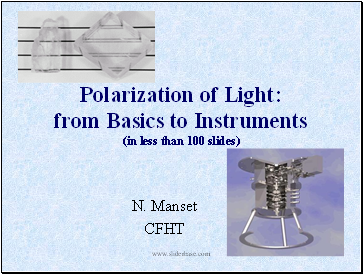Polarization of Light: from Basics to Instruments (in less than 100 slides)

N. Manset

CFHT

Slide 2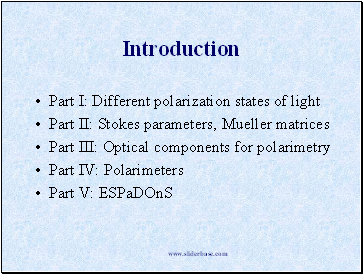## Introduction

Part I: Different polarization states of light

Part II: Stokes parameters, Mueller matrices

Part III: Optical components for polarimetry

Part IV: Polarimeters

Slide 3## Different polarization states of light

Light as an electromagnetic wave

Mathematical and graphical descriptions of polarization

Linear, circular, elliptical light

Polarized, unpolarized light

Slide 4Light as an electromagnetic wave

Light is a transverse wave,

an electromagnetic wave

Part I: Polarization states

Slide 5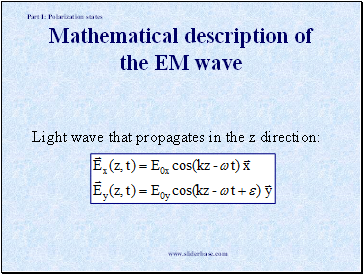Mathematical description of the EM wave

Light wave that propagates in the z direction:

Part I: Polarization states

Slide 6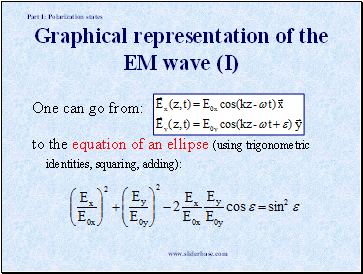Graphical representation of the EM wave (I)

One can go from:

to the equation of an ellipse (using trigonometric identities, squaring, adding):

Part I: Polarization states

Slide 7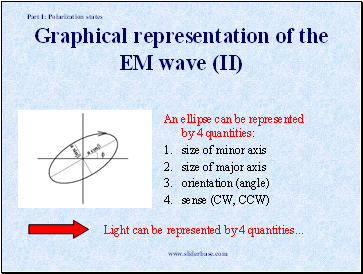Graphical representation of the EM wave (II)

An ellipse can be represented by 4 quantities:

size of minor axis

size of major axis

orientation (angle)

sense (CW, CCW)

Light can be represented by 4 quantities .

Part I: Polarization states

Slide 8Vertically polarized light

If there is no amplitude in x (E0x = 0), there is only one component, in y (vertical).

Part I: Polarization states, linear polarization

Slide 9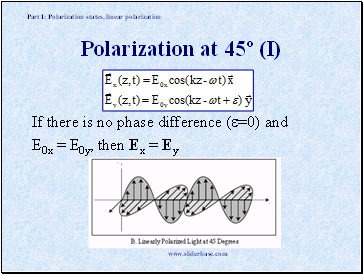Polarization at 45º (I)

If there is no phase difference (=0) and

E0x = E0y, then Ex = Ey

Part I: Polarization states, linear polarization

Slide 10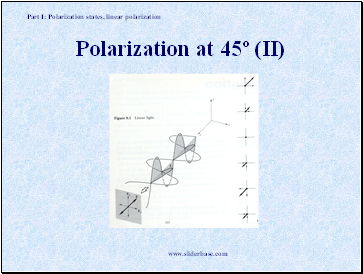Polarization at 45º (II)

Part I: Polarization states, linear polarization

Slide 11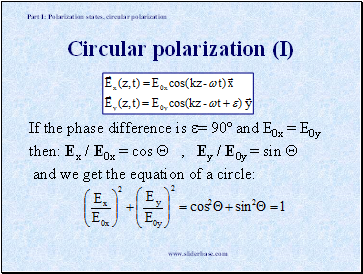Circular polarization (I)

If the phase difference is = 90º and E0x = E0y

then: Ex / E0x = cos  , Ey / E0y = sin 

and we get the equation of a circle:

Go to page:
1  2  3  4  5  6  7  8  9  10